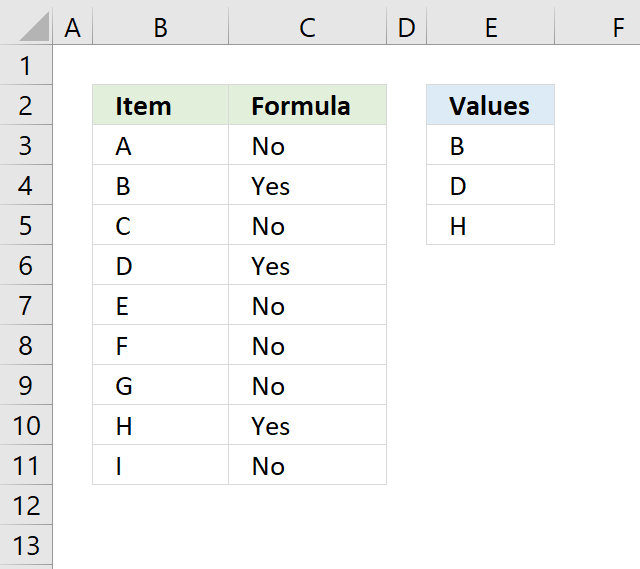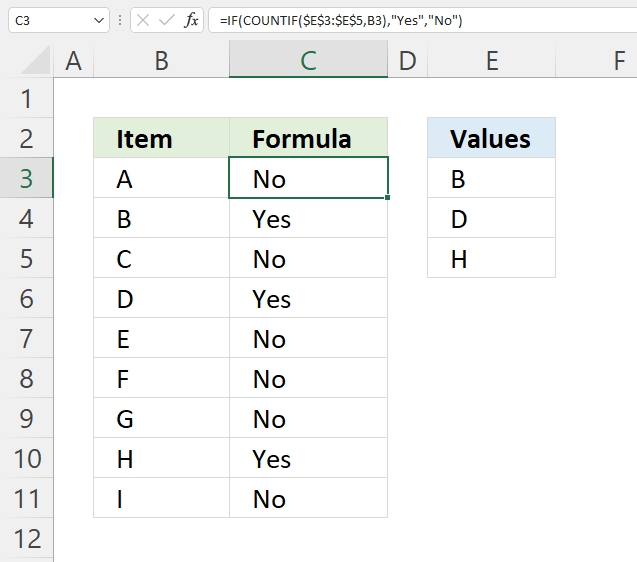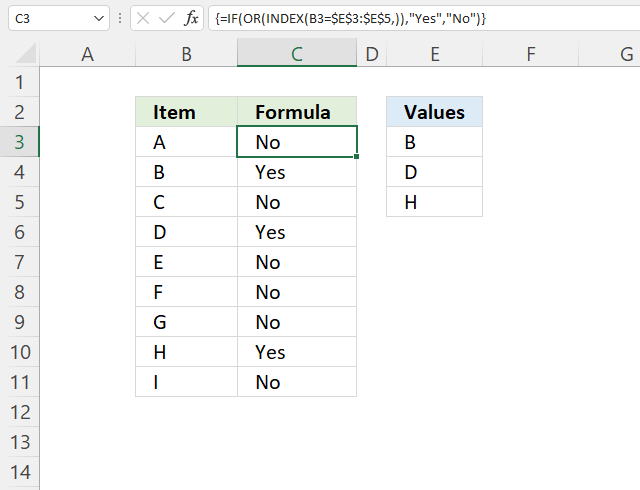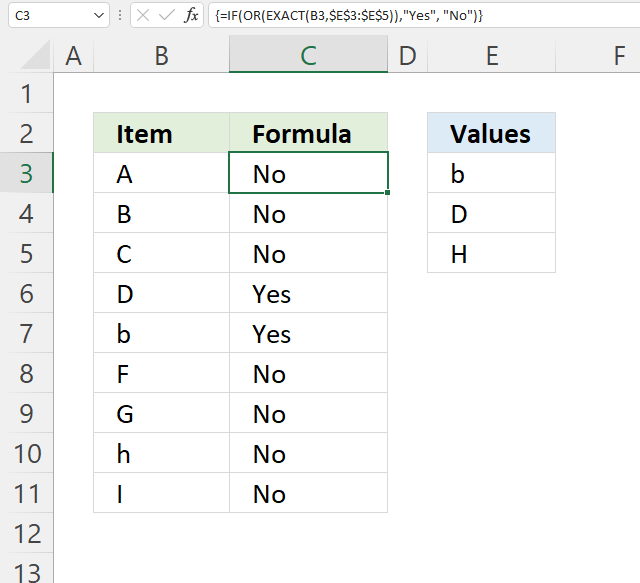Author: Oscar Cronquist Article last updated on November 02, 2021This article demonstrates formulas that check if a cell value is equal to any value in a given list.

## 1. If cell equals value from list - regular formulaFormula in cell C3:

=IF(COUNTIF(\$E\$3:\$E\$5,B3),"Yes","No")

### Explaining formula in cell C3

#### Step 1 - Count cells based on condition

The COUNTIF function counts how many values in E3:E5 match cell B3, it returns 0 (zero) since "A" is not found in E3:A5.

COUNTIF(rangecriteria)

COUNTIF(\$E\$3:\$E\$5,B3)

becomes

COUNTIF({"B";"D";"H"},"A")

and returns 0 (zero).

#### Step 2 - Count cells based on condition

The IF function then returns "YES" if the logical expression is 1 or more, "No" if the logical expression returns 0 (zero).

IF(logical_test, [value_if_true], [value_if_false])

IF(COUNTIF(\$E\$3:\$E\$5,B3),"Yes","No")

becomes

IF(0,"Yes","No") and returns "No" in cell C3.

## 2. If cell equals value from list - array formulaI recommend using the regular formula above, this array formula checks if cell B3 is equal to any of the values in E3:E5, the IF function returns Yes if one of the values is a match and No if none of the values match.

=IF(OR(B3=\$E\$3:\$E\$5),"Yes","No")

### 2.1 How to enter an array formula

To enter an array formula, type the formula in a cell and then press and hold CTRL + SHIFT simultaneously, now press Enter once. Release all keys.

The formula bar now shows the formula enclosed with curly brackets telling you that you entered the formula successfully. Don't enter the curly brackets yourself.

### 2.2 Explaining formula in cell C3

#### Step 1 - Compare values

The equal sign compares the value in cell B3 with each value in cell range E3:E5.

B3=\$E\$3:\$E\$5

becomes

"A"={"B";"D";"H"}

and returns

{FALSE; FALSE; FALSE}. If the cell matches a value in E3:E5 it returns TRUE, if not FALSE. In this case, all logical expressions return FALSE.

#### Step 2 - Evaluate OR function

The OR function returns TRUE if at least one argument is TRUE.

OR(logical1[logical2])

OR(B3=\$E\$3:\$E\$5)

becomes

OR({FALSE; FALSE; FALSE})

and returns FALSE. The OR function returns FALSE since all arguments are FALSE.

#### Step 3 - Evaluate IF function

The IF function returns "YES" if the logical expression is TRUE, "No" if the logical expression is FALSE.

IF(logical_test, [value_if_true], [value_if_false])

IF(OR(B3=\$E\$3:\$E\$5),"Yes","No")

becomes

IF(FALSE,"Yes","No")

and returns "No". The IF function then returns No in cell C3.

### Alternative regular formula

The INDEX function changes the above formula into a regular formula.

=IF(OR(INDEX(B3=\$E\$3:\$E\$5,)),"Yes","No")

The INDEX and SUMPRODUCT functions have this characteristic, you just have to know when and where to apply them.

### Related post

Use IF + COUNTIF to evaluate multiple conditions

The image above demonstrates a formula that matches a value to multiple conditions, if the condition is met the formula […]

## 4. If cell equals value from list case sensitiveFormula in cell C3:

=IF(OR(EXACT(B3, \$E\$3:\$E\$5)), "Yes", "No")

### 4.1 Explaining formula in cell C3

#### Step 1 - Identify equal values case sensitive

The EXACT function lets you compare values also considering upper and lower cases.

EXACT(text1, text2)

EXACT(B3, \$E\$3:\$E\$5)

becomes

EXACT("A", {"b"; "D"; "H"})

and returns

{FALSE; FALSE; FALSE}.

#### Step 2 - Evaluate OR function

The OR function returns TRUE if at least one argument is TRUE.

OR(logical1[logical2])

OR(EXACT(B3, \$E\$3:\$E\$5))

becomes

OR({FALSE; FALSE; FALSE})

and returns FALSE.

#### Step 3 - Evaluate IF function

The IF function returns "YES" if the logical expression is TRUE, "No" if the logical expression is FALSE.

IF(logical_test, [value_if_true], [value_if_false])

IF(OR(EXACT(B3, \$E\$3:\$E\$5)), "Yes", "No")

becomes

IF(FALSE, "Yes", "No")

and returns "No".

## 5. Get excel *.xlsx file

If cell equals value from list.xlsx